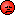Thread: IIF Statement Question View Single Post
 Bob BedellFriend of Wrox Join Date: Jun 2003 Location: , , USA. Posts: 1,093 Thanks: 1 Thanked 12 Times in 11 PostsI'm much confused, data types aside.

It should be impossible to test for a divide-by-zero condition using IIf. Take for example:

Sub Test(numerator As Integer, denominator As Integer)
Dim dblNew As Double

dblNew = IIf(denominator > 0, numerator / denominator, 0)

End Sub

' Test IIf
Call Test 1,0

IIf always evaluates both the truepart and the falsepart of the expression. So, the above would yield:

dblNew = IIf(0 > 0, 1/0, 0)

or try

dblNew = IIf(0 = 0, 0, 1/0)

You would think the first example would return the falsepart (0). But it doesn't. When IIf executes, the truepart (1/0) is also always evaluated, and raises a divide by zero error if the denominator is 0. So you can't check for divide-by-zero using IIf in VBA. You'll always get Run-time error 11: Divide by zero, if the value of the 'denominator' is zero; the falsepart isn't returned.

Then I ran the following in Jet SQL:

SELECT Num, PricePer, Cost, IIf([PricePer]>0,([Cost]/[PricePer]),0) AS Amt
FROM [Table]
WHERE Num=2;

This works fine. Why? It should fail if PricePer is 0. Are the VBA IIf function and the Jet SQL IIf function not the same function? Weird.

The work-around I've always used in VBA to test for divide-by-0 is:

Sub Test1(numerator As Integer, denominator As Integer)
Dim result As String

result = Divide(numerator, denominator)

'Value of result is "" if exit function executed
If result = "" Then
MsgBox "Divide by zero attempted"
Else
MsgBox result
End If
End Sub

Function Divide(n As Integer, d As Integer) As String

If d = 0 Then
Exit Function 'result = ""
Else
Divide = n / d
End If

End Function

Or I've called function Divide from a query. Can't figure out why IIf works when its embedded in SQL, but not in VBA.Bob